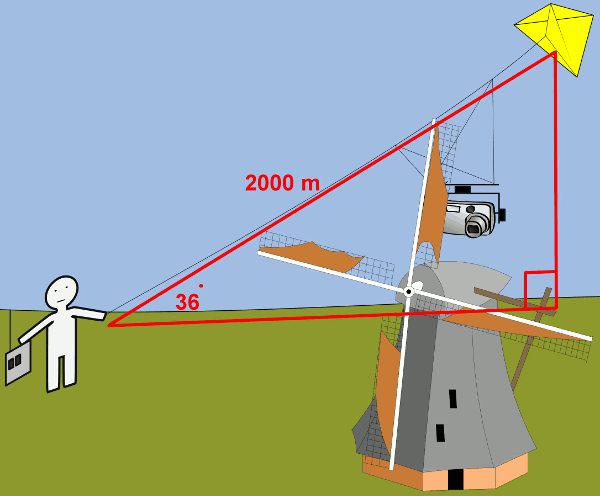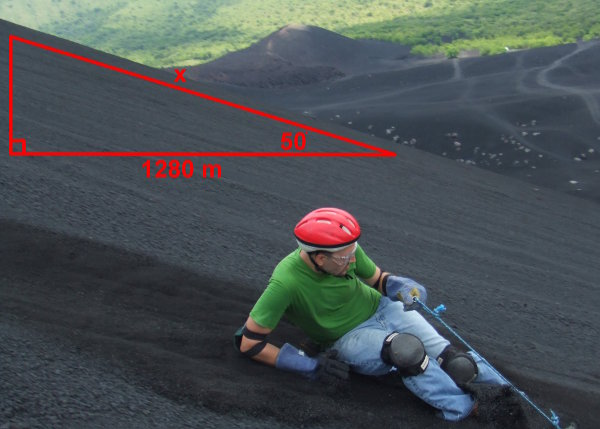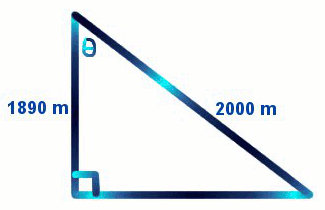# Trigonometry Cosine Rule

There are 3 trigonometry rules which are:

• Sin θ = O/H
• Cos θ = A/H
• Tan θ = O/A (when there is no hypotenuse)

## Example One - Cosine Rule to Find the Adjacent SideKite aerial photography uses remote control to move a kite and take camera shots from a bird's eye view. The length of the kite cable is 2000 metres. The angle that the cable makes with the ground is 36°. What is the distance in a horizontal direction that the kite is away from the photographer?

cos θ = A/H
(Always draw a diagram and write the rule. Then substitute the numbers and letters specific to this question. See the next line of working.)

cos 36 = x / 2000
(If x is on the top of the fraction, multiply both sides of the equation by the number on the bottom which is 2000.)

cos 36 × 2000 = x
(Now type cos 36 × 2000 on your calculator. You may need to close the bracket after the 36.)

x = 1618.03

The horizontal distance is 1618.03 metres.

## Question - Finding the Adjacent Side

The same weather balloon is being blown by a stronger sideways breeze. The angle that the cable makes with the ground is now 40°, but the cable length remains the same. What is the horizontal distance from the station now? (Draw a diagram.)

1532.09 metres

## Example Two - Cosine Rule to Find the HypotenuseIn Nicaragua in South America, volcano boarding is becoming a popular extreme sport. If the horizontal distance from the centre of the volcanic hill is 1280 metres and the angle of the slope with level ground is 50°, what is the length of the whole boarding slope?

cos θ = A/H
(Always draw a diagram and write the rule. Then substitute the numbers and letters specific to this question. See the next line of working.)

cos 50 = 1280/x
(If x is on the bottom of the fraction, multiply both side by x and divide both sides by cos 50. In effect, you will swap the sin and the side. See the next line of working.)

x = 1280 / cos 50
(Type 1280 / cos 50 on your calculator. You may need to close the bracket after the 50.)

x = 1991.33

The sloped length is 1991.33 metres.

## Question - Finding the Hypotenuse

After a recent volcanic eruption, there is more ash. The new horizontal distance is 1300 metres, but the angle of the slope remains the same. How much further can a surfer travel from top to bottom compared with before the eruption? (Draw a diagram.)

31.11 metres

## Example Three - Cosine Rule to Find an Angle

A weather balloon takes measurements of altitude (height above the ground), air temperature, humidity and oxygen levels at different altitudes. Because it contains expensive electronic devices, it is fixed by a long cable to a pole at the weather station. The altitude reading is 1890 metres and the length of cable is 2000 metres. What is the angle between the cable and the vertical?cos θ = A/H
(Always draw a diagram and write the rule. Then substitute the numbers and letters specific to this question. See the next line of working.)

cos θ = 1890 / 2000

θ = cos-1 (1890 / 2000)
(Type Shift Cos (1890/2000) on your calculator. Use brackets for the fraction part.)

θ = 19.09°

The angle is 19.09°.

## Question - Finding an Angle

In a breeze, the weather balloon has blown further sideways. Its altitude is 1500 metres, but the cable is the same. What is the angle between the cable and the vertical now? (Draw a diagram.)